# Lennard-Jones fluid

The simplest off-lattice model of the liquid is a system of spherical particles, interacting via a pairwise potential, that depends on the distance between them,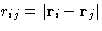. As we know, molecules repel, when they are very close to each other, but attract each other at larger separation. The most widely used model for such interactions is Lennard-Jones potential.   Off-lattice Monte-Carlo Simulations. Lennard-Jones Fluid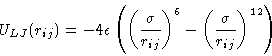(1)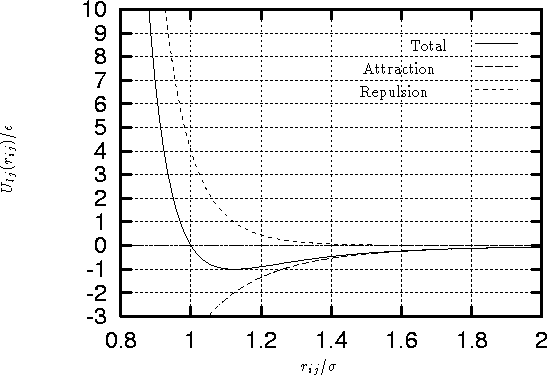It is a sum of the short-range repulsive contribution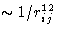and attractive contribution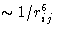.

Attractive term
describes the interaction between two molecules. When two atoms are brought close to each other, charge density fluctuations in the first atoms's electron shell induce polarization of the electron shell of the other. The attraction between the two induced dipoles leads to the attractive inverse sixth power term. Very similar interaction is observed between two colloid particles, or polyelectrolyte molecules, where the induced dipoles are due to the fluctuations of the counter-ion density distribution. These fluctuations and the induced electron shell polarization are described by very similar equations and therefore lead to the similar answer. But their nature is very different.

The first one is due to the quantum fluctuations of the electron shell interactions, the second is caused by the thermal fluctuations of colloid particles, or polyions. Lennard-Jones potential parameters should not depend on temperature, pressure, etc.

The functional form (1/r6) comes from the exact quantum-mechanical solution for the non-polar, neutral atoms with the spherically symmetric electron shell, like e.g. noble gas atoms.And therefore for them it is exact. It is nevertheless always used as approximation for other atoms.

Repulsive term
Unlike attraction, the exact functional form for the repulsive interaction is not well known and is approximated either by the exponential, or by the inverse power term. Inverse twelfth power is practical for the computer simulations, as it is the square of 6, and hence the energy can be calculated faster.

Parameters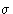and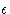As is seen from the figure 1, and equation 1,is a measure of the atom's diameter, it's the distance where the energy is zero. Minimum energy is at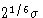, and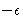is the minimal value of the energy. The values of this parameters are usually extracted from some experimental data, like crystal structures, thermodynamic properties, kinetic coefficients, etc. They are very hard to get from the first principles.Next: Periodic Boundaries for Off-Lattice Up: Off-lattice Monte-Carlo Simulations. Lennard-Jones Previous: Off-lattice Monte-Carlo Simulations. Lennard-Jones

© 1997 Boris Veytsman and Michael Kotelyanskii
Tue Nov 11 18:36:02 EST 1997